## Compress Signal

After you use Wavelet Signal Analyzer to examine the characteristics of the decomposition, you can use the app to compress the signal. You can either apply a global threshold to the entire decomposition, or independent thresholds to specific levels or terminal nodes. The app automatically reconstructs the compressed signal based on the thresholded coefficients. You can also compare statistics of the original and compressed signals.

To compress a scenario, click on the Analyzer tab. When you do so, the app:

• Enables the Threshold option in the Compression section. You can select one of these thresholding methods:

• Global Hard Thresholding — Apply the same threshold to all levels of the decomposition

• Level Dependent Hard Thresholding — Apply different thresholds to different levels of the decomposition

• Applies a default, nonzero threshold to the decomposition.

• Enables you to plot the thresholded coefficients. Select Thresholded Coefficients under Coefficients ▼.

• Updates the Retained Coefficients column in the Levels table. The column shows the number of coefficients used by the app to construct the compressed signal.

• Plots the compressed signal. The app also adds the name of the compressed signal in the format `scenarioName_compressed` to the plot legend.

• Updates any statistics plots which are open to include the corresponding plots for the compressed signal.When you enable compression, horizontal cursors appear in the plots of the decomposition coefficients. To apply a threshold to a decomposition level, you can either drag the horizontal cursor to a threshold or enter a value in the text field.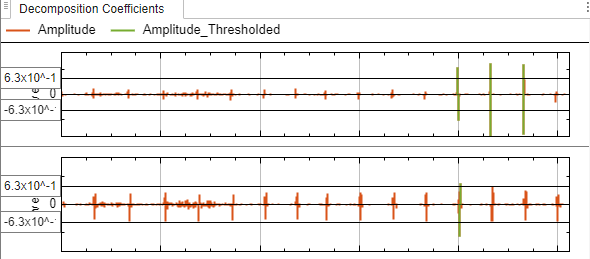• For global hard thresholding, when you apply a threshold in one level plot, the app automatically applies the same threshold in all level plots. For example, if you move a horizontal cursor in one plot, the cursors in all the plots move.

• For level-dependent hard thresholding, you can apply different thresholds in different level plots. For example, if you move a horizontal cursor in one plot, cursors in the other plots remain fixed.

• You can apply a threshold in all plot types: amplitude, magnitude, and sorted magnitude.

• For complex-valued signals, the app applies the thresholds to the coefficient magnitudes. For more information, see Wavelet Decomposition of Complex-Valued Signal Using Wavelet Signal Analyzer.

• To reset thresholds to the default value, disable and then re-enable compression.

Note

For global hard thresholding, the app applies the threshold to all coefficients in the decomposition, including the approximation coefficients. If you want to use all the approximation coefficients to construct the compressed signal, switch to level-dependent hard thresholding and set the threshold of the approximation coefficients to 0.

### Example: Compress Audio Signal

Load a recording of a female speaker saying "I saw the sheep". The sample rate is 22,050 Hz. The signal is in the workspace variable `sheep`, and the sample rate is in `fs`.

`load wavsheep`

Import the signal into Wavelet Signal Analyzer. Specify the sample rate in the app.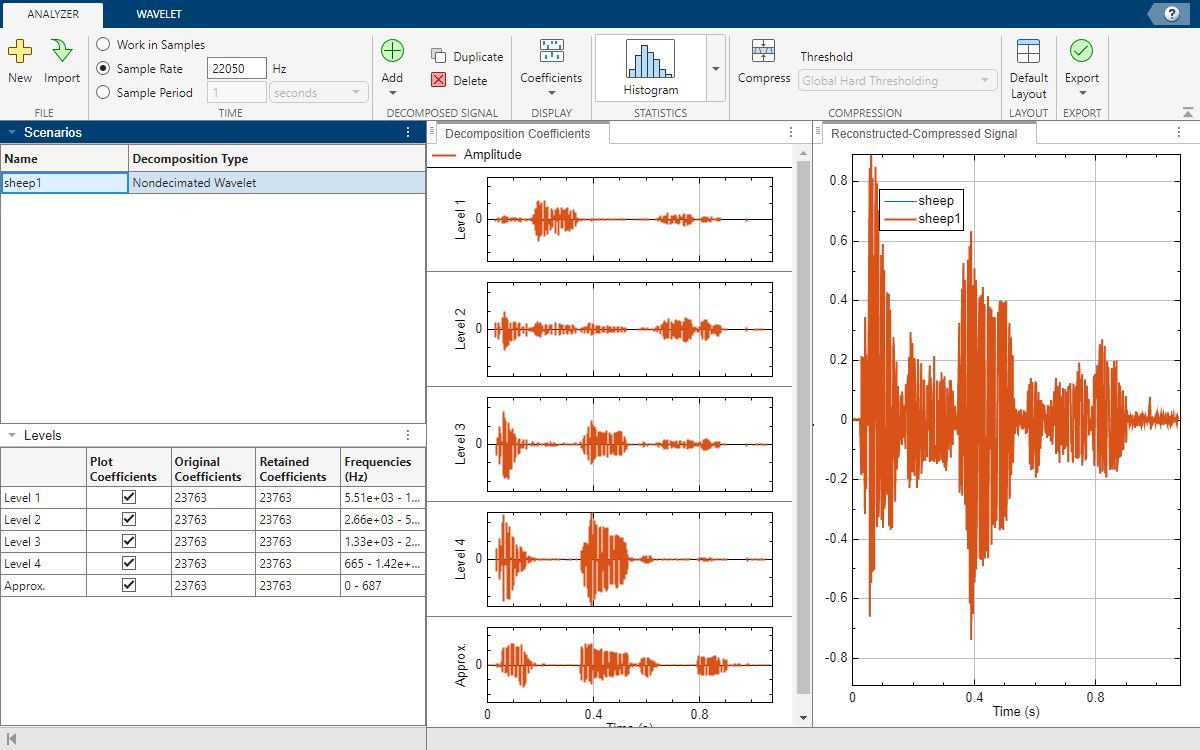Identify Decomposition Levels to Threshold

Plot the relative energies of the decomposition levels. In the Statistics section, click the arrow to open the gallery. Under For All Levels, click Energy by Level. Approximately 75% of the energy of the signal is concentrated in the approximation and level 4 coefficients.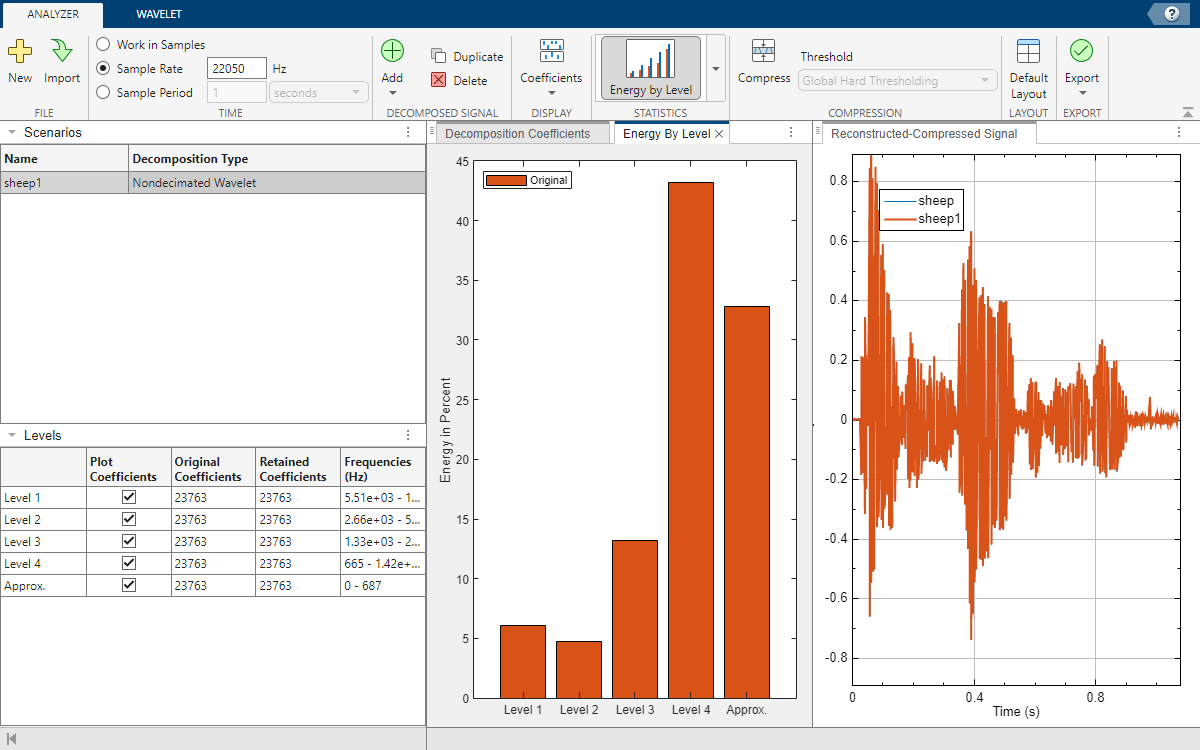Enable Compression

Switch to the Decomposition Coefficients pane. Plot the sorted magnitudes of the coefficients. In the Display section, click Coefficients ▼. Under Coefficients, click Sorted Magnitude. In the Compression section, click Compress.

• The default thresholding method is Global Hard Thresholding. The app applies the same default threshold to all decomposition levels. The app plots the coefficients that it used in the compressed signal in a different color.

• The app plots the compressed signal `sheep1_compressed` in a different color.

• The Retained Coefficients column in the Levels table displays the number of coefficients used by the app to construct the compressed signal.

To plot the thresholded coefficients, in the Display section, under Coefficients, click Thresholded Coefficients.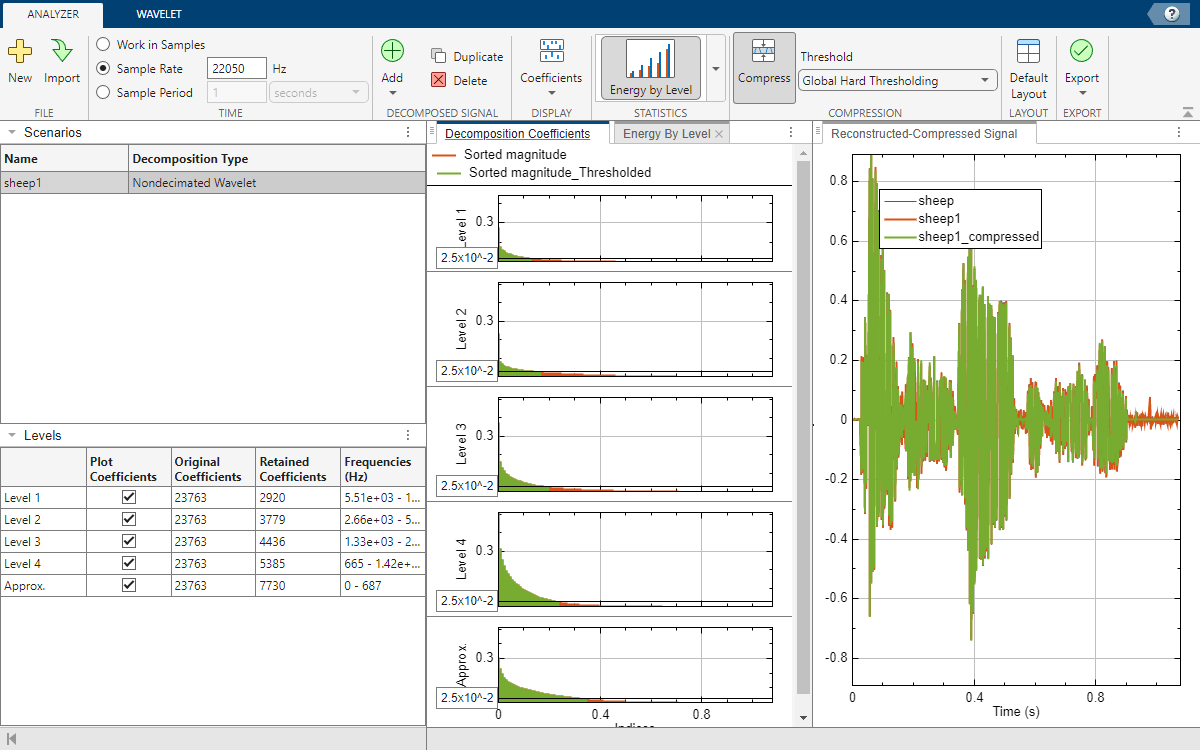Apply Thresholds

First, apply a global threshold of 0.1 to the decomposition. In any level coefficients plot, you can either drag the horizontal cursor to the threshold or enter `0.1` in the text field. The number of retained coefficients changes, and all the plots update.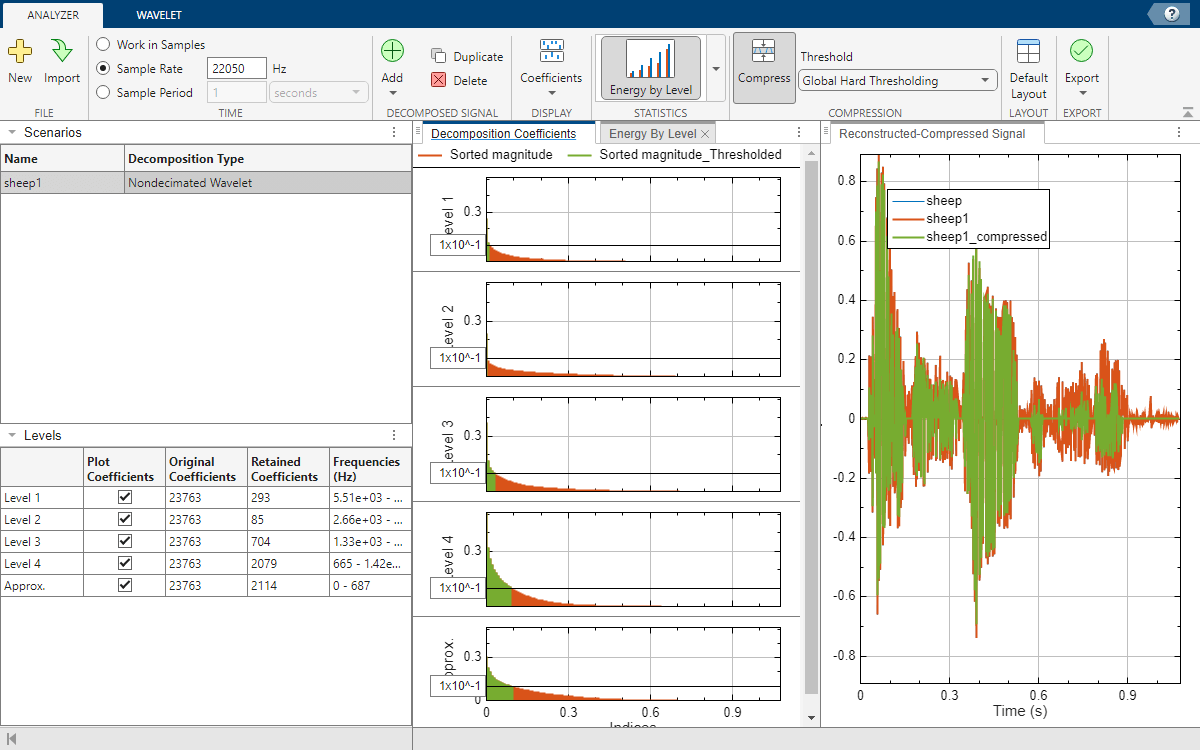Switch to the Energy By Level pane. The app plots the relative energies of the decompositions associated with the original and compressed signal.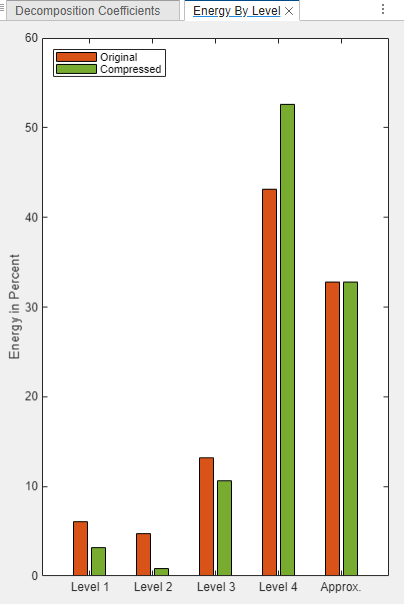In the original signal, the approximation and level 4 coefficients account for approximately 75% of the total energy of the signal. Perform level-dependent hard thresholding. Select Level Dependent Hard Thresholding under Threshold. For Levels 1, 2, and 3, set the threshold so that no coefficients are retained. For the level 4 and approximation coefficients, set the threshold to 0. The app uses only the approximation and level 4 coefficients to construct the compressed signal.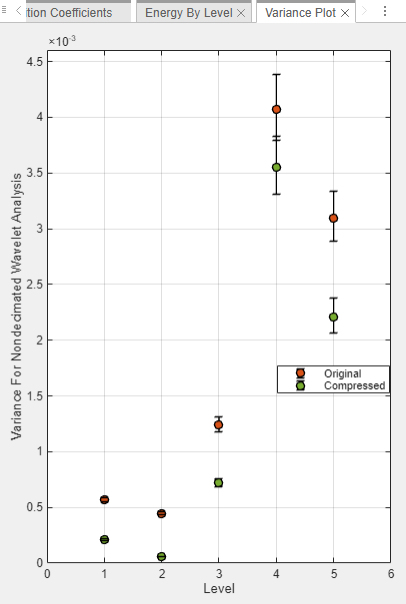The approximation and level 4 coefficients account for 100% of the total energy in the compressed signal.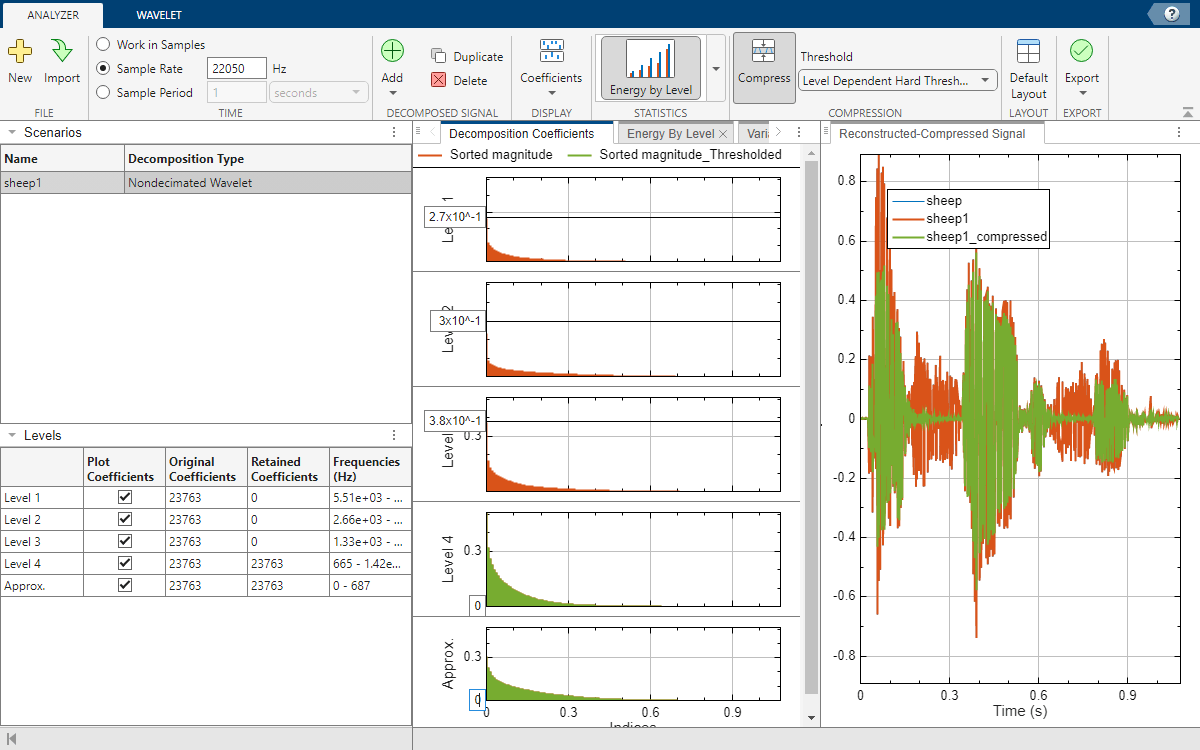Recreate Compressed Signal in Workspace

Click Export. Then, under Compressed Signal, select Generate MATLAB® Script. An untitled script opens in your editor with the following executable code. You can save the script as is or modify it to apply the same compression to other signals. Run the code.

```% Perform the decomposition using MODWT numberOfLevels = 4; wt = modwt(sheep,"sym4",numberOfLevels); % Compute the energy by level for the decomposition energyByLevel = 100*sum((wt.^2),2)/sum(wt.^2,"all"); % Thresholds for compressing the imported signal compressionThresholds = ... [0.5112 0.5112 0.5112 0 0]'; % Duplicate coefficients for thresholding wc = wt; % Apply threshold to coefficients for idx = 1:numel(compressionThresholds) thr = abs(compressionThresholds(idx)); w = wc(idx,:); w = wthresh(w,"h",thr); wc(idx,:) = w; end % Energy by level for the compressed signal energyByLevelForCompressed = 100*sum((wc.^2),2)/sum(wc.^2,"all"); % Reconstruct the compressed signal using IMODWT sheep1_compressed = imodwt(wc,"sym4");```

Play the original signal and the compressed signal. Pause for 3 seconds between playbacks.

```soundsc(sheep,fs) pause(3) soundsc(sheep1_compressed,fs)```

### Workflow

Previous StepCurrent StepNext Step
Explore Signal DecompositionCompress SignalShare Results